# Civil Engineering - Applied Mechanics

### Exercise :: Applied Mechanics - Section 3

6.

A body A of mass 6.6 kg which is lying on a horizontal platform 4.5 m from its edge is connected to the end of a light string whose other end is supporting a body of mass 3.2 kg as shown in below figure. If the friction between the platform and the body A is 1/3, the acceleration is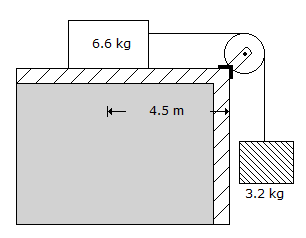A. 0.5 m/sec2 B. 0.75 m/sec2 C. 1.00 m/sec2 D. 1.25 m/sec2.

Explanation:

No answer description available for this question. Let us discuss.

7.

The motion of a particle is described by the relation x = t2- 10t + 30, where x is in metres and t in seconds. The total distance travelled by the particle from t = 0 to t = 10 seconds would be

 A. zero B. 30 m C. 50 m D. 60 m E. none of these.

Explanation:

No answer description available for this question. Let us discuss.

8.

Three forces which act on a rigid body to keep it in equilibrium. The forces must be coplanar and

 A. concurrent B. parallel C. concurrent parallel D. none of these.

Explanation:

No answer description available for this question. Let us discuss.

9.

A square hole is made in a circular lamina, the diagonal of the square is equal to the radius of the circleas shown in below figure the shift in the centre of gravity is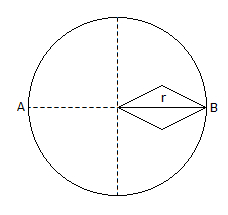A.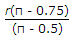B.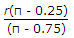C.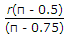D.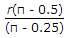Explanation:

No answer description available for this question. Let us discuss.

10.

For perfectly elastic bodies, the value of coefficient of restitution is

 A. zero B. 0.5 C. 1.0 D. between 0 and 1.